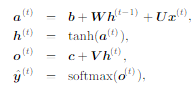# Potentially incorrect re-implementation of RNN in PyTorch tutorial

## Problem

I am going over the this PyTorch tutorial on PyTorch official website, which tries to classify names into their origins without using `torch.nn.RNN` but uses basic building block `torch.nn.Linear` instead (as could be seen here.

``````class RNN(nn.Module):
def __init__(self, input_size, hidden_size, output_size):
super(RNN, self).__init__()

self.hidden_size = hidden_size

self.i2h = nn.Linear(input_size + hidden_size, hidden_size)
self.i2o = nn.Linear(input_size + hidden_size, output_size)
self.softmax = nn.LogSoftmax(dim=1)

def forward(self, input, hidden):
combined = torch.cat((input, hidden), 1)
hidden = self.i2h(combined)
output = self.i2o(combined)
output = self.softmax(output)
return output, hidden

def initHidden(self):

``````

As much as I find this implementation quite insightful, I found its formulation inconsistent with formal definitions found in Goodfellow’s deep learning book (Page 374)..
Specifically,

• There is no tanh() applied to updated hidden state.
• Output state is from combined vector instead of updated hidden state.

However, according to this tutorial, the result does seem to be good. I am not sure if there is formal name for this architecture.

Could someone help me? Thank you in advance.

There is no tanh() applied to updated hidden state.

If you use nn.RNN(), it uses tanh() as a default activation function even if it is not specified by the user.

Output state is from combined vector instead of updated hidden state.

I guess the tutorial is incorrect in terms of its implementation where the output and the new hidden state are different because they are derived from independent weights(i2h, i2o). As you can see in the code snippet below, the result of hidden state and the last sequence output should be the same

``````import torch.nn as nn
rnn = nn.RNN(10, 10, batch_first=True)

inp = torch.randn((1,3,10))

result = rnn(inp)

print(result)
print(result)
``````

which has the same result of

``````tensor([[[-0.0253,  0.5289, -0.2582, -0.5125, -0.4577,  0.5456, -0.4042,